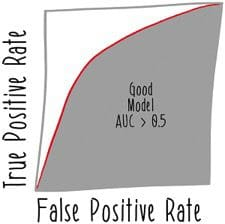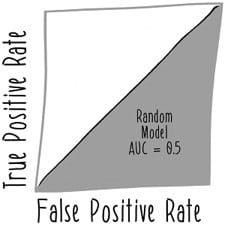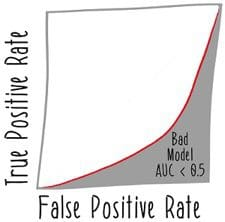# Machine Learning Model Metrics

In this article we explore how to calculate machine learning model metrics, using the example of fraud detection. We'll see lots of different ways that we can try to understand just how good our learned model is.

MLlib has some nice built-in functionality to calculate the ROC curve for our binary classifier (Listing 5).

Listing 5. Training Performance Summary

```val trainingSummary = model.summary (1)

val binarySummary = trainingSummary.asInstanceOf[BinaryLogisticRegressionSummary] (2)

val roc = binarySummary.roc (3)

roc.show() (4)

```

1. Producing the summary of the model’s performance
2. Casting that summary to the appropriate type, BinaryLogisticRegressionSummary
3. The ROC curve for the model
4. Printing the ROC curve for inspection

The model summary is relatively new functionality within MLlib, so it's not available for all classes of models. There are also limitations to its implementation, such as the one that requires you to use asInstanceOf to cast the summary to the correct type. Make no mistake, using asInstanceOf like this is bad Scala style; it represents a subversion of the type system. But MLlib is still being rapidly developed, so this cast operation is just a sign of an incomplete implementation within MLlib. Development on MLlib is very active, but machine learning is an enormous domain for any one library to support. New functionality is being added at a rapid pace and the overarching abstractions are being dramatically improved. Look for rough edges like this class cast to disappear in future versions of Spark.

Of course, we're building massively scalable machine learning systems that operate largely autonomously. So, who has time to look at a graph and make a decision about what constitutes a good enough model? Well, one of the uses of an ROC curve is to get a single number about the performance of a model, the area under the ROC curve. The higher this number, the better the model's performance. You can even make strong assertions about a model's utility using this calculation. Remember that a random model would be expected to perform according to the line x = y on the ROC curve. The area under that line would be 0.5, so any model with an area under the curve of less than 0.5 can safely be discarded as being worse than a random model.

Figures 3, 4, and 5 show the differences in the area under the curve of a good, random, and worse than random model.Listing 6 shows the implementation of validating for performance better than random.

Listing 6. Validating Training Performance

```def betterThanRandom(model: LogisticRegressionModel) = { (1)
val trainingSummary = model.summary (2)

val binarySummary = trainingSummary.asInstanceOf[BinaryLogisticRegressionSummary] (3)

val auc = binarySummary.areaUnderROC (4)

auc > 0.5 (5)
}

betterThanRandom(model) (6)
```

1. Defining a function to validate that a model is better than random
2. The training summary
3. Class casting
4. The area under the ROC curve
5. Testing if the area under the curve is greater than a random model
6. An example call to validate a model

This validation can serve as a very useful safety feature in a machine learning system, preventing you from publishing a model that could be deeply detrimental. In the Kangaroo Kapital example, since fraud is so much rarer than normal transactions, a model that failed this test would very likely be falsely accusing a lot of angry animals of fraud for normal use of their credit cards.

This technique can be extended beyond basic sanity checks like this. If you record the historical performance of your published models, you can compare the performance of your newly trained models to them. Then a logical validation would be to not publish a model with meaningfully different performance than the current published model. We'll discuss some more techniques for model validation a bit later.

We're not done asking questions about our model yet. There are other model metrics that we can consider. The metrics that we have seen thus far try to capture an aspect of a model's performance. In particular, it's not hard to imagine a model that does a bit better on precision but not on recall or vice versa. An F measure (or sometimes F1 score) is a statistic that tries to combine the concerns of precision and recall in the same metric. Specifically, it is the harmonic mean of the precision and the recall. Listing 7 shows two ways of formulating the F measure.

Listing 7. F Measure

```F measure = 2 * (precision * recall) / (precision + recall)

F measure = (2 * true positives) / (2 * true positives + false positives + false
negatives)

```

Using the F measure as a model metric may not always be appropriate. It trades off precision versus recall evenly, which may not correspond to the modeling and business objectives of the situation. But it does have the advantage of being a single number which can be used to implement automated decision making.

For example, one use of the F measure is to set the threshold that a logistic regression model uses for binary classification. Internally, a logistic regression model is actually producing probabilities. To turn them into predicted class labels, we'll need to set a threshold to divide positive (fraud) predictions from negative (not fraud) predictions. Figure 6 shows some example prediction values from a logistic regression model and how they could be divided into positive and negative predictions using different threshold values.While the F measure is not the only way of setting a threshold, it's a useful one, so let's see how to do it. Listing 10 shows how to set a threshold using the F measure of the model on the training set.

Listing 8. Setting a Threshold Using the F Measure

```val fMeasure = binarySummary.fMeasureByThreshold (1)

val bestThreshold = fMeasure.where(\$"F-Measure" === maxFMeasure) (3)

model.setThreshold(bestThreshold) (4)
```

1. Retrieving the F measure for every possible threshold
2. Finding the maximum F measure
3. Finding the threshold corresponding to the maximum F measure
4. Setting that threshold on the model

Now the learned model will use the threshold selected on the basis of F measure to distinguish between positive and negative predictions.

Summary

In this article we've explored how to calculate machine learning model metrics, using the example of fraud detection. We've seen lots of different ways that we can try to understand just how good our learned model is.